Multiplication of matrices

Chapter 3 Class 12 Matrices
Concept wise

To multiply two matrices,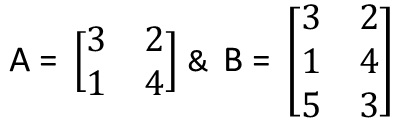We first write their order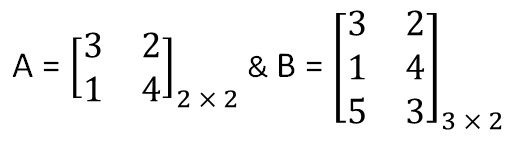For multiplication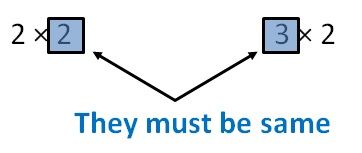Since 2 ≠ 3

We cannot multiply them

But, if we multiply BA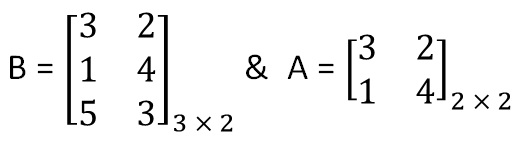Then,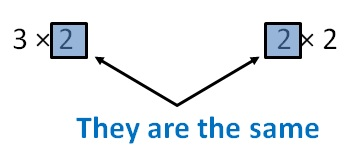So, order of matrix after multiplication is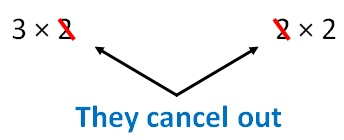= 3 × 2

Let’s learn how to multiply them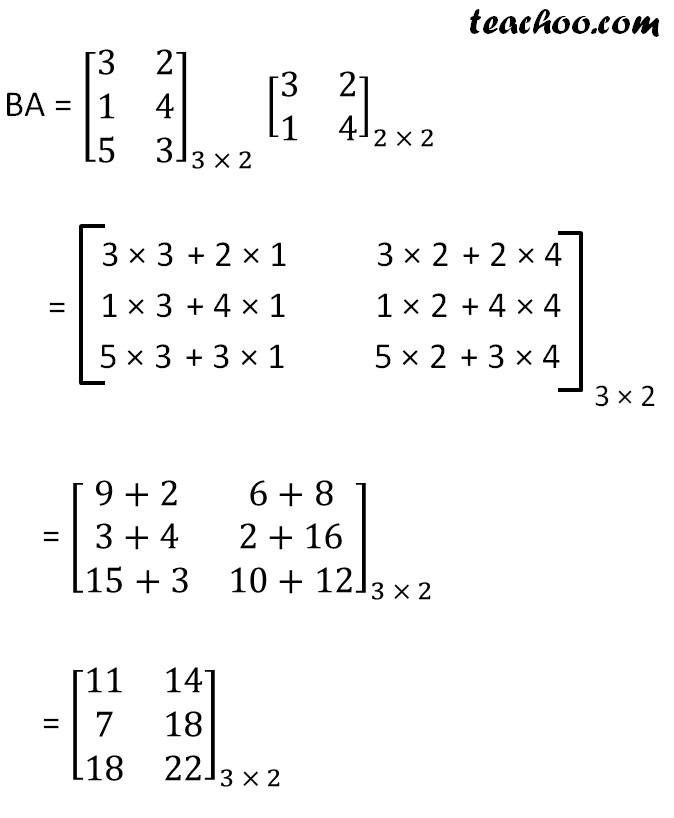So,

AB was not possible, but BA was possible

Thus, AB ≠ BA

Let’s do some more examples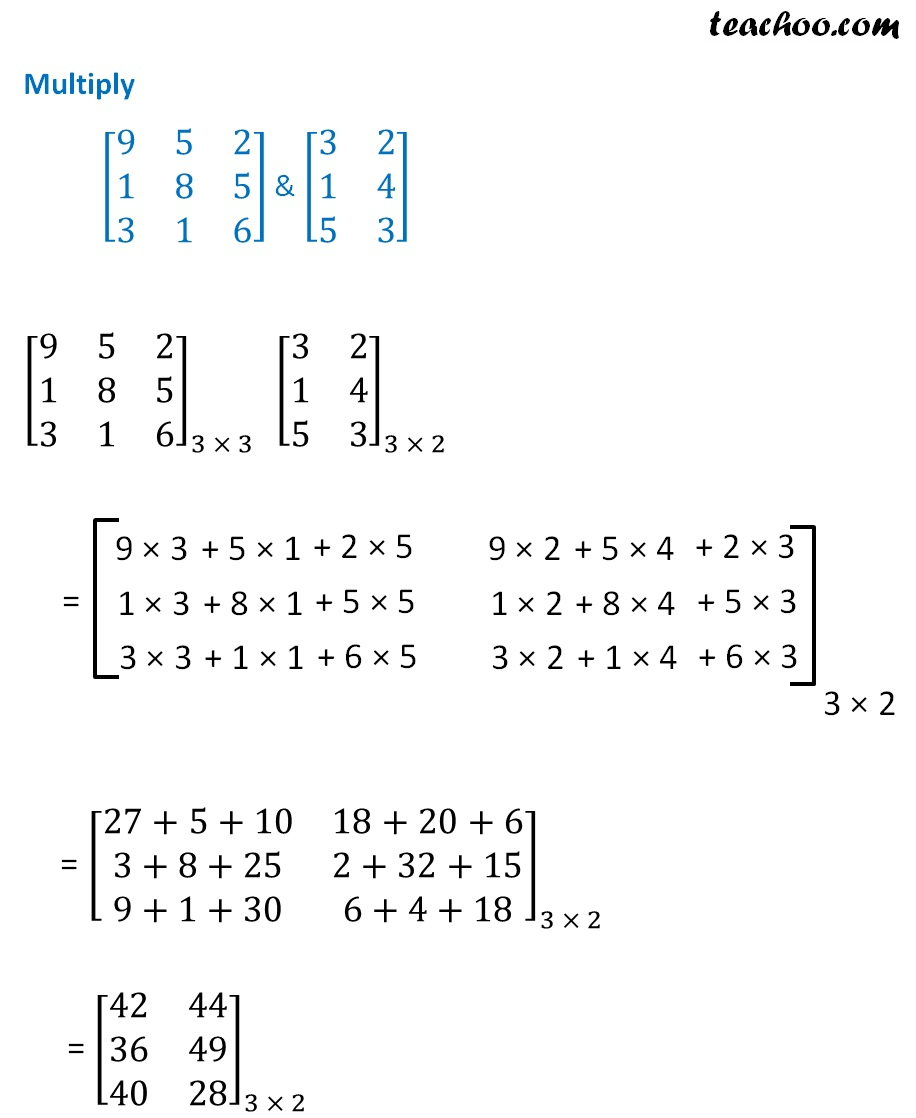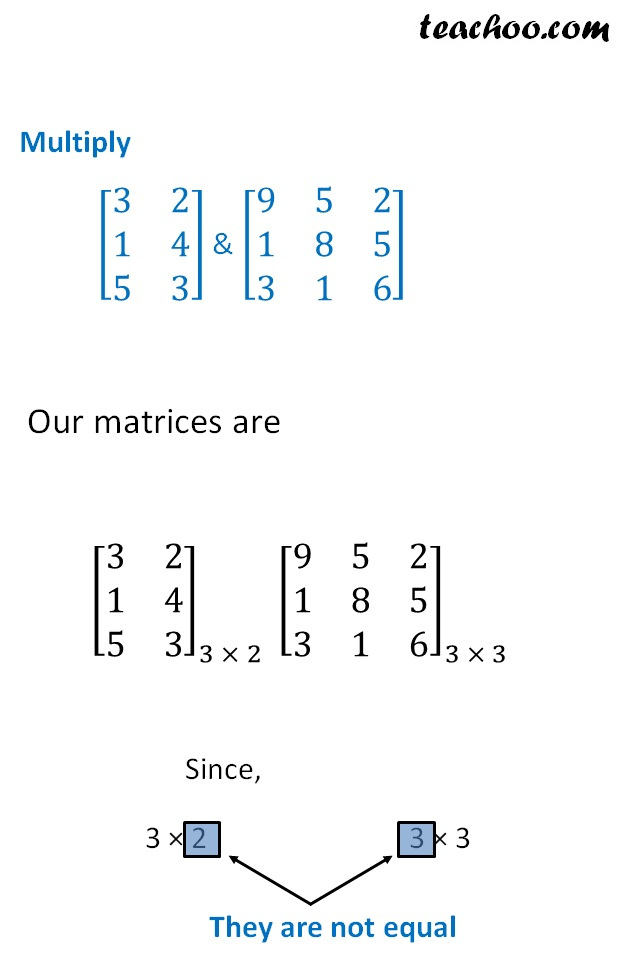So, multiplication is not possible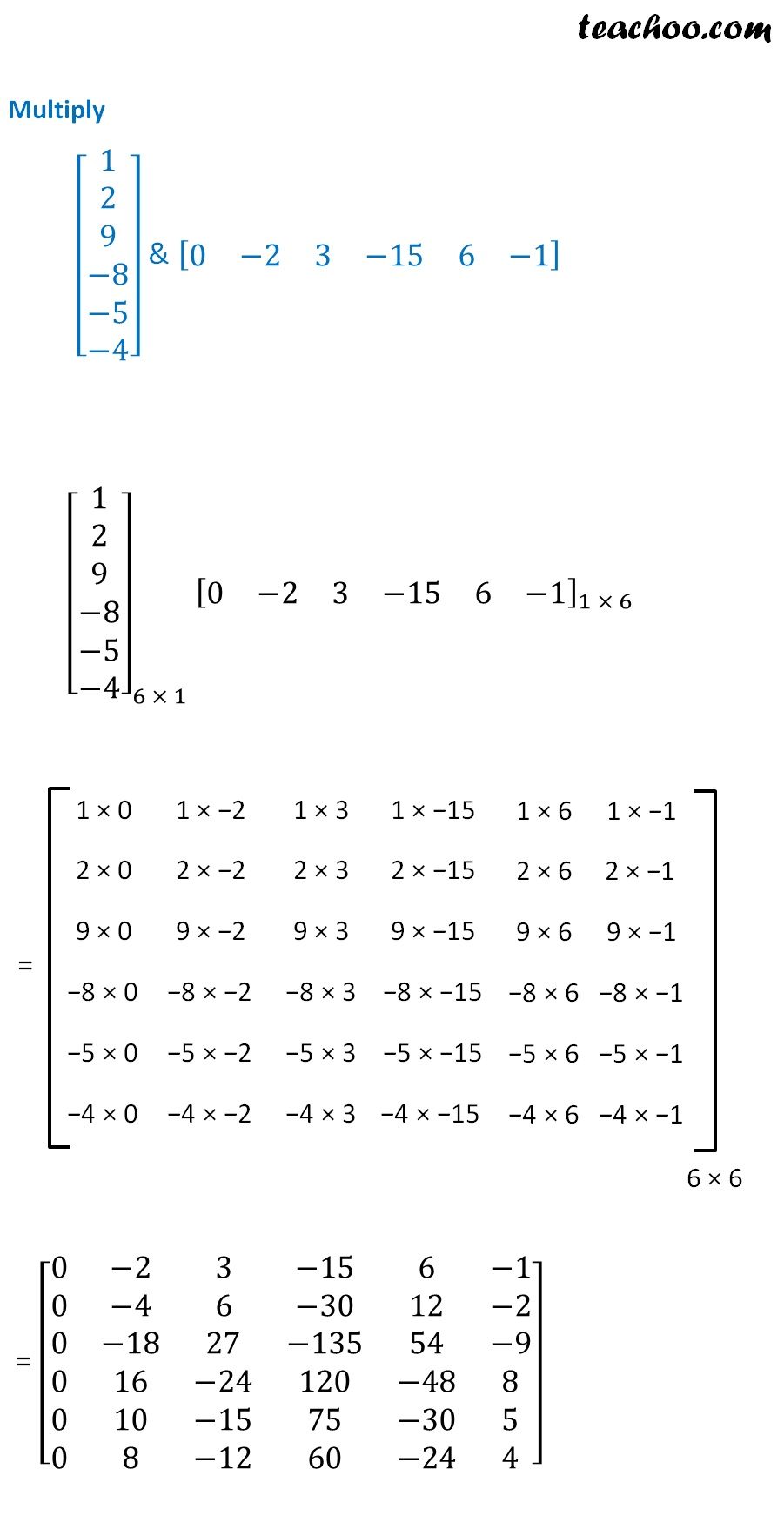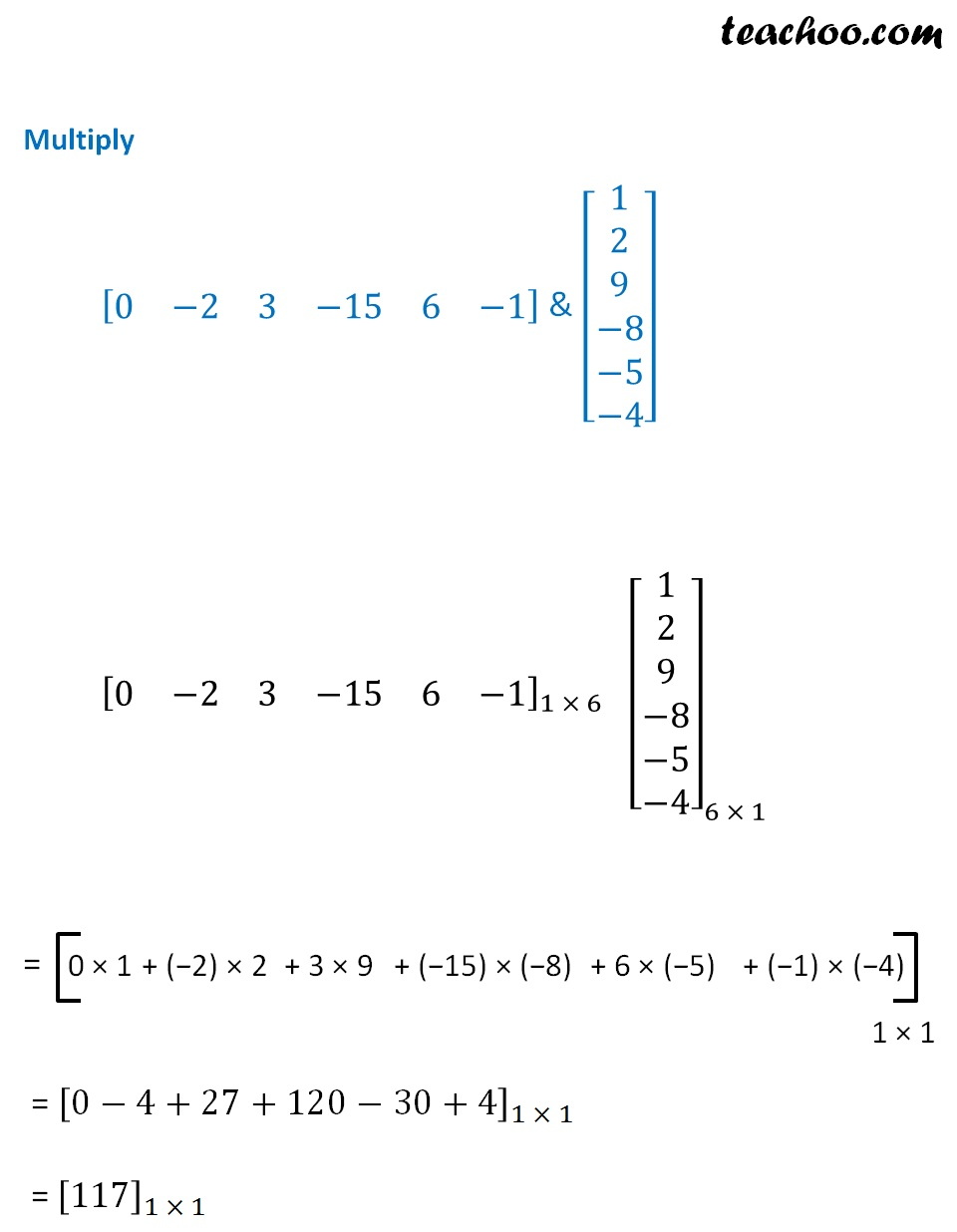Learn in your speed, with individual attention - Teachoo Maths 1-on-1 Class

### Transcript

A = [■8(3&2@1&4)] & B = [■8(3&2@1&4@5&3)] A = [■8(3&2@1&4)]_(2 × 2) & B = [■8(3&2@1&4@5&3)]_(3 × 2) For multiplication 2 × 2 3 × 2 i.e B = [■8(3&2@1&4@5&3)]_(3 × 2) & A = [■8(3&2@1&4)]_(2 × 2) 3 × 2 2 × 2 3 × 2 2 × 2 They cancel out BA = [■8(3&2@1&4@5&3)]_(3 × 2) [■8(3&2@1&4)]_(2 × 2) 3 × 3 + 2 × 1 1 × 3 + 4 × 1 = [■8(9+2&6+8@3+4&2+16@15+3&10+12)]_(3 × 2) [■8(11&14@7&18@18&22)]_(3 × 2) = [■8(42&44@36&49@40&28)]_(3 × 2) Multiply [■8(3&2@1&4@5&3)] & [■8(9&5&2@1&8&5@3&1&6)] Our matrices are [■8(3&2@1&4@5&3)]_(3 × 2) [■8(9&5&2@1&8&5@3&1&6)]_(3 × 3) Since, They are not equal Multiply [■8(1@2@9@−8@−5@−4)] & [■8(0&−2&3&−15&6&−1)] [■8(1@2@9@−8@−5@−4)]_(6 × 1) [■8(0&−2&3&−15&6&−1)]_(1 × 6) = [■8(0&−2&3&−15&6&−1@0&−4&6&−30&12&−2@0&−18&27&−135&54&−9@0&16&−24&120&−48&8@0&10&−15&75&−30&5@0&8&−12&60&−24&4)] Multiply [■8(0&−2&3&−15&6&−1)] & [■8(1@2@9@−8@−5@−4)] [■8(0&−2&3&−15&6&−1)]_(1 × 6) [■8(1@2@9@−8@−5@−4)]_(6 × 1) = [0−4+27+120−30+4]_(1 × 1) =〖 〗_(1 × 1)